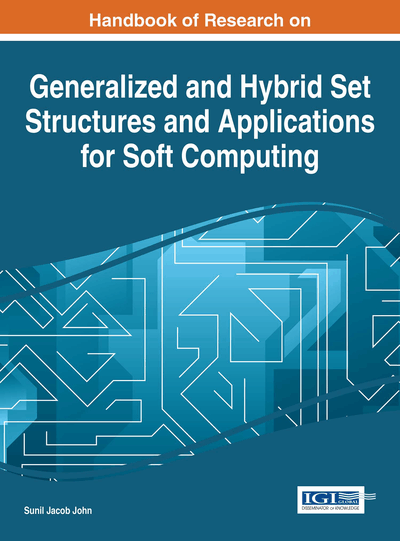# On Theory of Multisets and Applications

B. K. Tripathy (VIT University, India)
DOI: 10.4018/978-1-4666-9798-0.ch001

## Abstract

Although multiple occurrences of elements are immaterial in sets, in real life situations repetition of elements is useful. So, the notion of multisets (also called as bags) was introduced, where repetition of elements is taken into account. Fuzzy set, intuitionistic (a misnomer here as intuitionistic mathematics has nothing to do with its fuzzy counterpart) fuzzy sets, rough sets and soft sets are extensions of the basic notion of sets as they model uncertainty in data. Following this multisets have been extended to fuzzy multisets, intuitionistic fuzzy sets, rough multisets and soft multisets. Many properties of basic sets have been extended to the context of multisets, fuzzy multisets, intuitionistic fuzzy sets, rough multisets and soft multisets. Several applications of different multisets mentioned above are found in literature. In this chapter, it is our aim to introduce the different concepts of multisets, their properties, current status and highlight their applications.
Chapter Preview
Top

## 1. Introduction

The notion of sets introduced by G.Cantor as a well-defined collection of distinct and distinguishable elements, does not permit repetition of elements and the order of occurrence of elements in it is immaterial. But, in many real life situations we find that the repetition of elements is very important. For example, simply stating the different kinds of vegetables in a bag or basket does not provide detail idea of the vegetables unless we specify the number of vegetables of each kind in it. Similarly, if we state the kinds of scoring strokes like fours, sixes, twos, singles and threes of a batsman, it cannot provide the actual runs scored by him unless we specify the number of each kind of strokes. Many such examples can be found in real life. The earliest instance of use of multisets is found in the famous work,” Was sind und was sollen die Zahlen” meaning “The nature and meaning of numbers” of Richard Dedekind (The famous mathematician who also introduced the idea of Dedekind cuts and by the provided an alternate definition of real numbers) in the year 1888.An elaborative account of this is provided by Blizard (Blizzard, 1989). The word “multiset” was coined by N.G.de Bruijn and was communicated to Knuth in a private communication (Knuth, 1981). The next attempt to define a multiset independently dates back to 1971, when Cerf et al introduced it (Cerf et al, 1971). Also, we find that it was independently introduced by Peterson [Peterson, 1976]. Also, it was termed as bag and introduced by Yager (Yager, 1986). There are many alternate names for the same notion like heap, bunch, bag, sample, weighted set, occurrence set and fire set. In real life situations multisets are useful structures in many places in Mathematics and Computer Science. As we see in the prime number theorem, the prime factors can have multiplicities beyond one. So, in order to represent the prime factors of a given number can only be represented by a multi set but not a set. The roots of a monic polynomial over complex numbers is naturally can be represented by a multiset. As far as computer science is concerned the terminal string of a non-circular context free grammar forms a multiset. Multisets were used for proving termination properties and in some search and sort algorithms. Other notable uses of multisets are in the theory of Petri nets by Peterson (Peterson, 1976), relational databases by Yager (Yager, 1986), automata theory by Eilenberg and software verification in (Piskac et al, 2012).

## Key Terms in this Chapter

Fuzzy Set: One of the earliest uncertainty based models proposed by L.A.Zadeh in 1965 that assigns graded memberships to elements instead of binary membership provided by Crisp sets.

Rough Set: It is another efficient model to capture impreciseness proposed by Z.Pawlak in 1982, which follows the idea of G.Frege by defining the boundary region to capture uncertainty. It approximates every set by a pair of crisp sets, called the lower and upper approximations.

Multiset: This notion has an alternate name called as “bag”. It is based on the idea that unlike sets a collection can have multiple occurrences of elements.

Soft Set: It is one of the latest uncertainty based models introduced by Molodtsov in 1999, which introduces the notion of parameters in defining the membership of elements.

Intuitionistic Fuzzy Set: It is an uncertainty based model proposed by K.T.Attanasov in 1986, which extends the notion of fuzzy sets by relaxing the constraint in fuzzy sets that the non-membership value is one’s complement of the membership value of every element.

## Complete Chapter List

Search this Book:
Reset